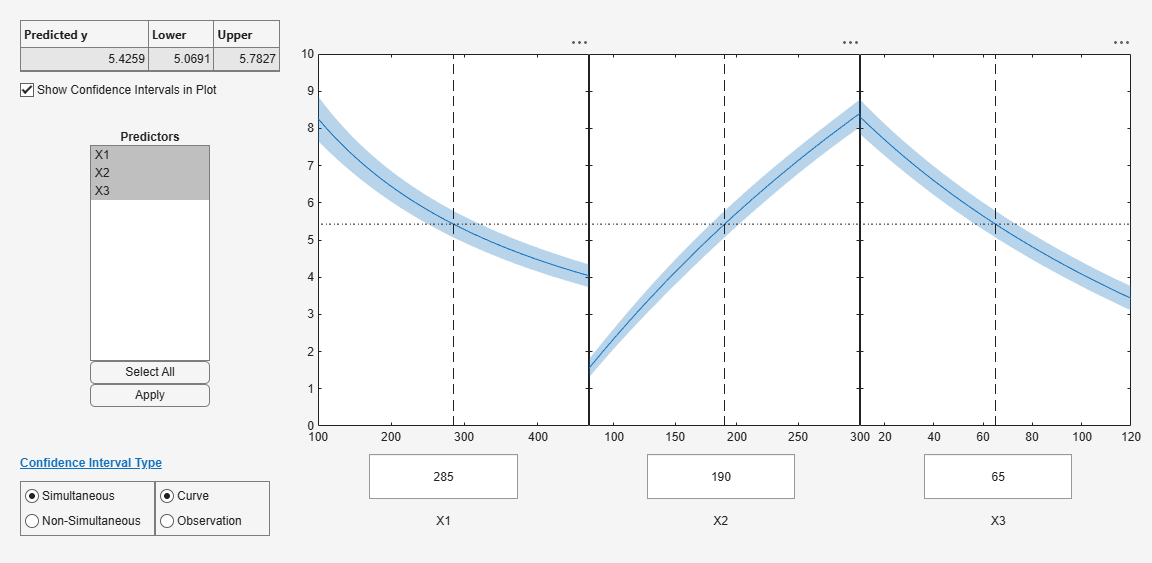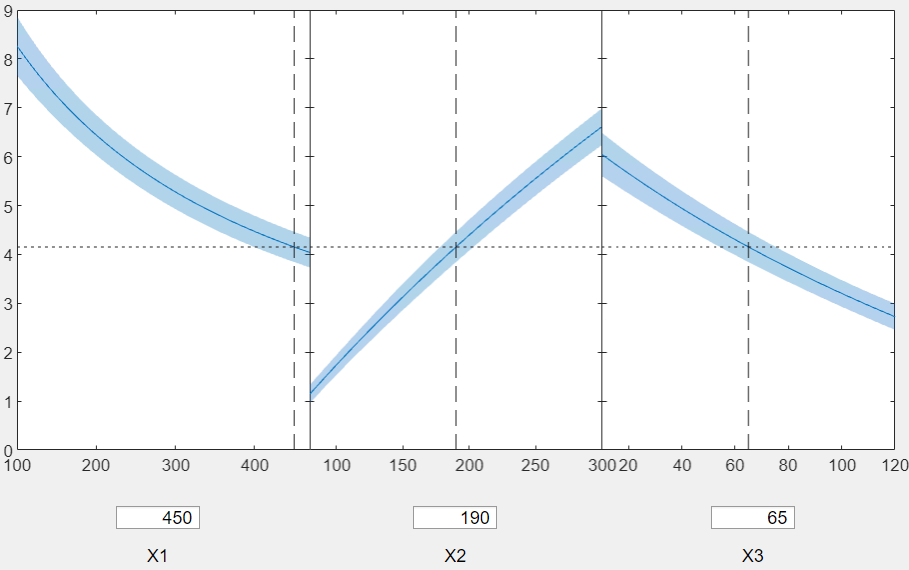plotSlice

Class: NonLinearModel

Plot of slices through fitted nonlinear regression surface

plotSlice(mdl)

Description

plotSlice(mdl) creates a new figure containing a series of plots, each representing a slice through the regression surface predicted by mdl. For each plot, the surface slice is shown as a function of a single predictor variable, with the other predictor variables held constant.

Input Arguments

 mdl Nonlinear regression model, constructed by fitnlm.

Examples

expand all

Plot slices of a fitted nonlinear model.

Load the reaction data and fit a model of the reaction rate as a function of reactants.

mdl = fitnlm(reactants,rate,@hougen,[1 .05 .02 .1 2]);

Create a slice plot.

plotSlice(mdl)Drag the X1 prediction line to the right, and observe the change in the predicted response y and in the predicted response curves to X2 and X3.Tips

• If there are more than eight predictors, plotSlice selects the first five for plotting. Use the Predictors menu to control which predictors are plotted.

• The Bounds menu lets you choose between simultaneous or non-simultaneous bounds, and between bounds on the function or bounds on a new observation.